﻿ 某乘用车座椅E-NCAP鞭打性能仿真优化与试验对比

# 某乘用车座椅E-NCAP鞭打性能仿真优化与试验对比Simulation Optimization and Test Comparison of E-NCAP Whiplash Performance for a Passenger Car Seat

Abstract: As a passenger car is to be exported to Europe, its seat design standard must meet E-NCAP. In the FEA analysis stage, the seat headrest is optimized for low whiplash scores. The finite element model is established in the ANSA and the LS-DYNA software is used as the solver to simulate the seat model to improve the FEA whiplash score. The object is carried out according to the latest data state in order to meet the E-NCAP standard, and the whiplash performance will be further optimized according to the comparison results.

1. 引言

2. E-NCAP鞭打试验法规分析解读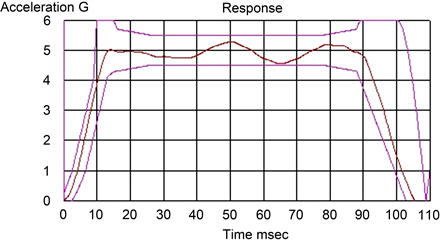Figure 1. E-NCAP low severity pulse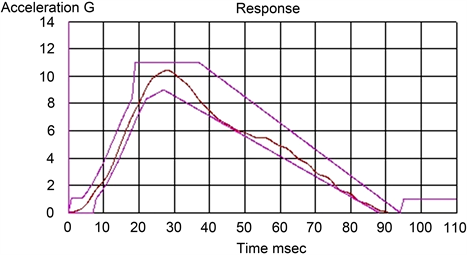Figure 2. E-NCAP medium severity pulse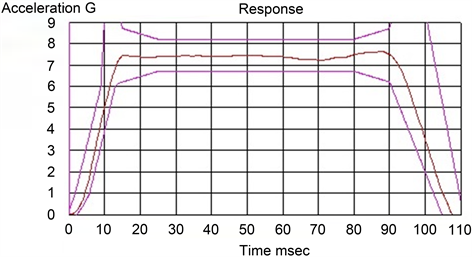Figure 3. E-NCAP high severity pulse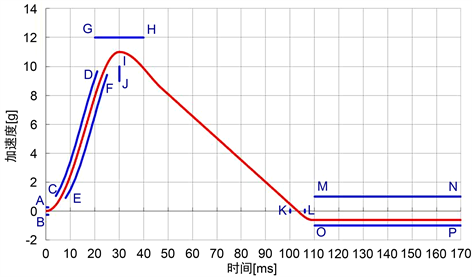Figure 4. C-NCAP acceleration pules

E-NCAP鞭打试验的评分方法主要是动态的高中低三条波形各3分，其中每个参数0.5分，在参数T1加速度和接触时间T-HRC中取分值大的进行计算，因此动态评价共计9分，还有2分的静态评价，因此总分数为11分，E-NCAP鞭打试验总体评分表和评价指标如表1所示。Table 1. 2018 Version E-NCAP whiplash general scoring table

3. 座椅鞭打模型建立和仿真结果分析

3.1. FEA模型建立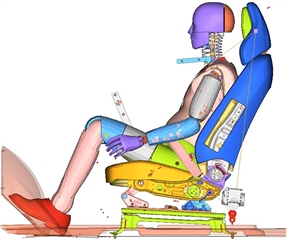Figure 5. Whiplash FEA model

3.2. FEA仿真结果Table 2. FEA Whiplash score table

${N}_{ep}\left(t\right)=\frac{{F}_{xp}\left(t\right)}{{F}_{xp-\mathrm{int}}}+\frac{{M}_{ye}\left(t\right)}{{M}_{ye-\mathrm{int}}}$

${N}_{ea}\left(t\right)=\frac{{F}_{xa}\left(t\right)}{{F}_{x-\mathrm{int}}}+\frac{{M}_{ye}\left(t\right)}{{M}_{ye-\mathrm{int}}}$

${N}_{fp}\left(t\right)=\frac{{F}_{xp}\left(t\right)}{{F}_{x-\mathrm{int}}}+\frac{{M}_{yf}\left(t\right)}{{M}_{yf-\mathrm{int}}}$

${N}_{fa}\left(t\right)=\frac{{F}_{xa}\left(t\right)}{{F}_{x-\mathrm{int}}}+\frac{{M}_{yf}\left(t\right)}{{M}_{yf-\mathrm{int}}}$

3.3. FEA仿真结果

1) 在有限元模型中量取假人头部和头枕之间的距离为30.3 mm，现调整模型将距离30.3 mm调整为21 mm，具体如图6所示。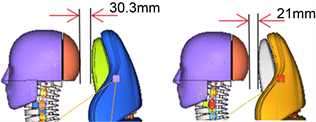Figure 6. Model optimization scheme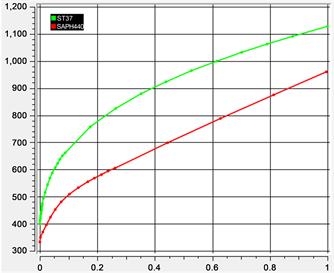Figure 7. Material stress-strain curve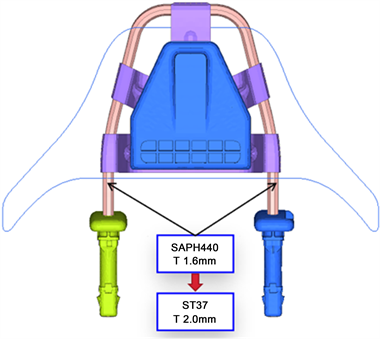Table 3. Comparison of FEA scores before and after optimization

4. 试验结果验证Figure 9. Physical test setup

Table 4. Comparison of physical test scores before and after optimization

5. 结语

 刘高, 王敏. 基于DOE的座椅鞭打试验优化分析[J]. 汽车实用技术, 2019(9): 146-148.

 王晋, 赵秀强, 王志涛, 梁宇, 乔鑫. 基于汽车座椅鞭打试验的仿真模型改进[J]. 汽车技术, 2015(9): 23-26.

 杨运生, 张晓龙, 娄磊. 基于台车试验的不同国家鞭打试验评价体系的相关性[J]. 汽车安全与节能学报, 2012, 3(2): 123-128．

 C-NCAP管理规则[M]. 天津: 中国汽车技术研究中心, 2018.

 李铁柱, 鲁后国, 阚洪贵. 基于仿真分析的某座椅鞭打性能改进[J]. 汽车技术, 2015(7): 18-21.

 符大兴, 刘华官, 李登云, 于国超. 乘用车座椅鞭打试验性能研究与改进[J]. 汽车实用技术, 2019(16): 110-114.

 胡远志, 胡源源, 刘西, 廖高健. 基于LS-DYNA的某座椅鞭打性能分析和优化[J]. 重庆理工大学学报(自然科学), 2017, 31(6): 8-14.

 吕宝锋, 韩峰, 岳国辉. 某轿车座椅防挥鞭伤性能改进[J]. 北京汽车, 2017(3): 40-42.

 European New Car Assessment Programme (Euro NCAP). Version 3.3.

Top